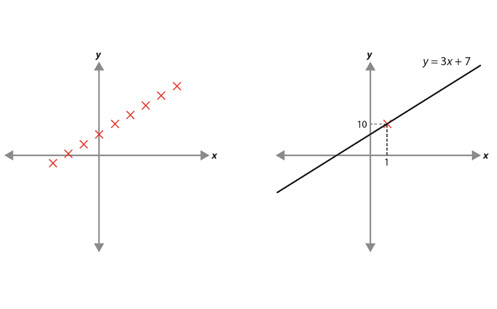# Solving linear equations (Years 7 and 8)

This paper explores the process of solving linear equations. It provides details of the knowledge students should have and some of the misconceptions they may hold. Examples given illustrate what these misconceptions may look like, how to address them, and support teachers in developing pedagogical and content knowledge.

Year level(s) Year 7, Year 8
Purpose Content knowledge
Keywords linear equation, solve

## Curriculum alignment

Strand and focus Algebra
Topics Linear relationships
AC: Mathematics content descriptions
ACMNA194 Solve linear equations using algebraic and graphical techniques. Verify solutions by substitution
ACMNA179 Solve simple linear equations